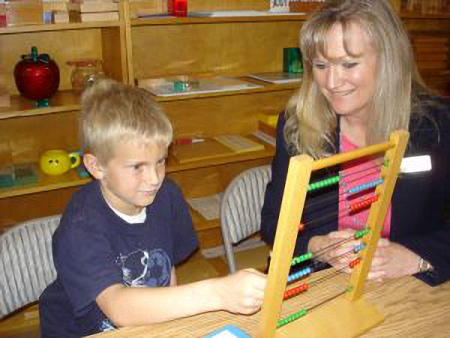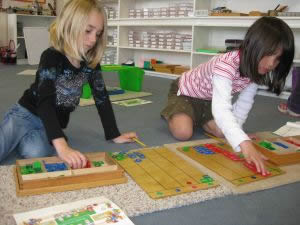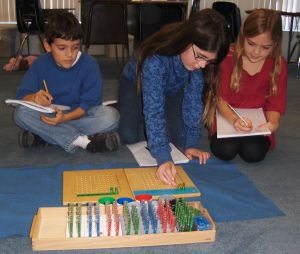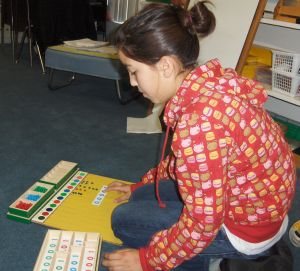# MathematicsOur elementary math program is extensive and can only be touched on briefly in this overview.  It begins with an introduction to place value and the four basic operations, using such concrete materials as the Golden Beads and the Stamp Game.  More advanced work in the four basic operations uses such materials as the Bead Frames for addition, subtraction and multiplication, the

Checkerboard for multiplication and Test Tubes for division.  The use of concrete materials for learning math provides the child with an opportunity to fully understand the inner workings of the operations (e.g. regrouping and exchanging).  Students in the 6 to 9 program also learn the associative, distributive and commutative properties, use materials to explore binomials and trinomials, powers of numbers,Subtraction Snake Game

squaring, square analysis, passage from one square to another and the Decanomial.  They are also introduced to fractions, fraction equivalencies, multiples, Lowest Common Multiple, Greatest Common Divisor, divisibility, and all four operations in fractions with like and unlike denominators.  Mastery of basic math facts is emphasized.  Children also work withmoney, standard and metric measurement and word/story problems.Elementary Math

Children in the 9 to 12 program continue to work in the four basic operations, moving always toward abstraction. Previous math facts mastery plays a crucial role.  They are also introduced to decimals and the four operations with decimals, on such concrete materials as the Yellow Decimal Board and the Decimal Checkerboard.  They begin work with integers, eventually doing all four operations.  They continue their study of binomials and trinomials, squaring and the powers of numbers.  They also learn expanded and exponential notations, square root, cubing, and cube root, ratio and proportion.  All mathematical processes are initially presented and practiced with concrete materials.  As the child gains understanding in the processes, he or she will move into abstracting them, with the ultimate goal of being fully competent in abstract operations by the end of the 6 to 12 program.Elementary Math Stamp GameElementary MathElementary Math